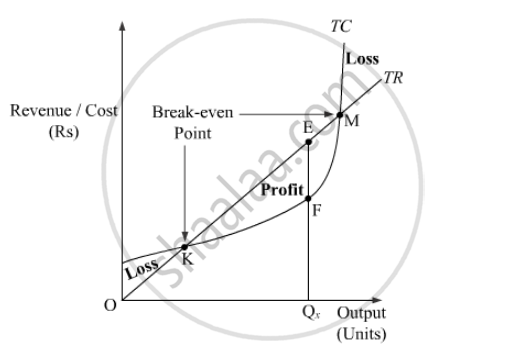Advertisement Remove all ads

# What is Meant by Producer’S Equilibrium? Explain the Conditions of Producer’S Equilibrium Through the ‘Total Revenue and Total Cost’ Approach. Use Diagram. - Economics

Advertisement Remove all ads
Advertisement Remove all ads
Advertisement Remove all ads

What is meant by producer’s equilibrium? Explain the conditions of producer’s equilibrium through the ‘total revenue and total cost’ approach. Use diagram.

Advertisement Remove all ads

#### Solution

Producer’s Equilibrium is a situation when a producer tries to maximise his profits. It is also assumed that he does not hold any stock of output produced, i.e. he sells whatever he produces.

Producer’s Equilibrium by TR-TC Approach:

According to the TR-TC approach, a producer attains the equilibrium at a point where the difference between the Total Revenue (TR) and the Total Cost (TC) curve is the maximum. At this equilibrium point, the monopolist maximises his profits.

The short-run equilibrium situation using the TR-TC approach is explained below diagrammatically.In the figure, the Total Revenue curve and the Total Cost curve of a producer are depicted by the TR and TC curves, respectively.

The profit for the producer is given by the vertical distance between the TR curve and the TC curve. It can be seen that this distance is the maximum at point E, where, the firm attains the equilibrium. The profits earned by the producer at this point equal the vertical distance EF. Accordingly, the equilibrium level of output is OQeAt the points M and K, the TR curve intersects the TC curve. Thus, at these points the producer earns zero-profits. These points are also known as the Break-Even points. To the left of the point K and to the right of the point MTC curve is above the TR curve. This implies that at these output levels, the producer is earning losses.

Concept: Conditions of Consumer's Equilibrium Using Marginal Utility Analysis
Is there an error in this question or solution?

#### APPEARS IN

Advertisement Remove all ads
Share
Notifications

View all notifications

Forgot password?